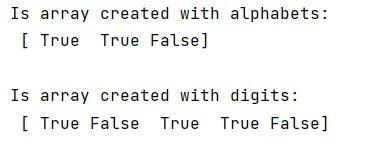# NumPy string functions like isalpha and isdigit

Learn about the NumPy string function similar like isalpha and isdigit.
Submitted by Pranit Sharma, on March 10, 2023

## Introduction

In NumPy string, the functions like isalpha and isdigit are numpy.char.isalpha() and numpy.char.isdigit(), which are used to check whether a given character is an alphabet or a digit.

## Python | numpy.char.isalpha() and numpy.char.isdigit() Functions

A string is a group of characters, these characters may consist of all the lower case, upper case, and special characters present on the keyboard of a computer system. A string is a data type and the number of characters in a string is known as the length of the string.

The numpy.char.isalpha() is used to check whether each element of the input array is an alphabet or not. It returns True if elements are alphabets and there is at least one character in the array.

On the other hand, numpy.char.isdigit() is used to check whether each character of an array is a digit or not. It returns True if the element of the array is a digit otherwise False.

Both methods just require a single parameter i.e., a which is the input array on which the test is done.

Let us understand with the help of an example,

## Python program to demonstrate the example of numpy.char.isalpha() and numpy.char.isdigit()

```# Import numpy
import numpy as np

# Check for alphabets

# Display result
print("Is array created with alphabets:\n",np.char.isalpha(arr),"\n")

# Check for digit
arr = ['123', '123abc', '9', '14', 'VIII']

# Display result
print("Is array created with digits:\n",np.char.isdigit(arr),"\n")
```

### OutputLanguages: » C » C++ » C++ STL » Java » Data Structure » C#.Net » Android » Kotlin » SQL
Web Technologies: » PHP » Python » JavaScript » CSS » Ajax » Node.js » Web programming/HTML
Solved programs: » C » C++ » DS » Java » C#
Aptitude que. & ans.: » C » C++ » Java » DBMS
Interview que. & ans.: » C » Embedded C » Java » SEO » HR
CS Subjects: » CS Basics » O.S. » Networks » DBMS » Embedded Systems » Cloud Computing
» Machine learning » CS Organizations » Linux » DOS
More: » Articles » Puzzles » News/Updates